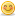1. ##IBM Aptitude papers

hello friends!
can u plz send me aptitude papers as i have exams in 4 days...Reply With Quote

2. ## Re: IBM Aptitude papers

A person has Rs 100/- in his pocket, he can as 25 pencils or 15books.He kept 15&#37; of the money for travelling expenses and purchased 5 pencils.So how many books he can purchase with the remaining money. a) 4 b) 3 c) 5 d)noneReply With Quote

3. ## Re: IBM Aptitude papers

A square plot of some size , at four corners equal squares of some size are cut and is formed as open box. If this open box carries 128ml of oil. What is the size of the plate i.e. side a.17 b.14 c.13 d.noneReply With Quote

4. ## Re: IBM Aptitude papers

Hi Akshay,

Its So simple....

a)He can't buy any books with the remaning money....

bcoz...

25 p = 100 ,

so each p=4
15 b = 100, so each b=100/15

Now, he has kept aside 15&#37;, that is 15 Rs

So, remaining is (5*4) + X * (100/15) + 15 = 100

X * (100/15) = 100 - 15 -20, so,

X = 65 * 15 / 100 = 39/4 ~ 9

v have l*b*h=128,

l=b(//Square plt),

So h become 2...

Then...

l=8+2+2=12

&& One thing..

Why dont u start a new thread???
plz post questions with a new thread....

Thanks & Regards,
Riju.Reply With Quote

5. ##Re: IBM Aptitude papers

traveling expenses=Rs 15
cost of 1 pencil=Rs 4
cost of 5 pencils=Rs 20
Money left =100-(15+20)= 65
Now, as in Rs 100 we can have 15 books
in Re 1, we can have 15/100 books
in the money left i.e 65 Rs we can have 15/100*65= 9.75
So person can buy 9 books and rest of the money he can keep with himselfReply With Quote

6. ##Re: IBM Aptitude papersReply With Quote

7. ## Re: IBM Aptitude papers

d) is the correct aswer.
post some tricky problems...Reply With Quote

8.

####Posting Permissions

• You may not post new threads
• You may not post replies
• You may not post attachments
• You may not edit your posts
•